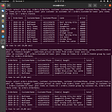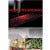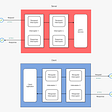# Hands-On with REDIS | Part 2

Welcome to this blog. We shall be looking at variety of examples to interact with Redis through Redis-CLI. So, Let’s get started.

Part #1 : Working with Strings.

Example #1.) We can ingest the <Key,Value> pair into the Redis and if the value is an Integer, then we can increment and decrement the same as well. In below example, the Key is “address” and value is 453.

`set <KEY> <VALUE>incr <KEY>`

Example #2.) We can also increment the integer value by a fixed number as well :-

`incrby <KEY> <VALUE_WITH_WHICH_INCREMENT_TO_BE_DONE>`

Example #3.) We can also decrement the integer value by a single number :-

`decr <KEY>`

Example #4.) We can also decrement the integer value by a fixed number as well :-

`decrby <KEY> <VALUE_WITH_WHICH_DECREMENT_TO_BE_DONE>`

Example #5.) We have to mandatorily have the value within double-quotes, if it’s a multi-word value :-

`set <KEY> <VALUE>`

Now, since the value is a multi-word value, we would set the same like this :-

The same can also be inquired from the Redis as follows :-

`get <KEY>`

Example #6.) Let‘s take another example of setting a value for yet another key. In below example, the keyName is “firstName” and value being set is “Manny”.

`set <KEY> <VALUE>`

Example #7.) There is yet another command with the help of which can first get the value first and immediately set the new value. We first get the value and then set the new value.

`getset <KEY> <VALUE>`

Example #8.) We can also set, multiple <Key, Value> pairs into the Redis as follows.

`mset <KEY_1> <VALUE_1> <KEY_2> <VALUE_2> <KEY_3> <VALUE_3>`

Here following keyNames and values have been set now :-

• <street, “seaward”>
• <city, “ventura”>
• <country, “usa”>
• <zip, “92101–2878292”>

Example #9.) We can also check from Redis, whether a particular key exists or Not. Also, we can delete the keys from Redis as follows :-

`exists <KEY>del <KEY>`

Example #11.) We can also expire the particular key from the Redis. Here “zip” is the KeyName and we want it to expire after 5 seconds.

`expire <KEY> <TIME_IN_SECONDS>`

Example #11.) Say suppose, we wanted to index a new <Key, Value> pair into the Redis and set an expiry timer @ that moment itself. In below example, “zip” is the key and timer for the same is 10 seconds.

`set <KEY> <VALUE> ex <TIME_IN_SECONDS>`

Now, if we check for this key, after 10 seconds, the same shall not be found in Redis :-

Part #2 : Working with Hash Data-Structure.

Example #1.) The Value in the Redis, itself can also be a HashMap. This is the case of nested maps.

`hmset <KEY> <INNER_KEY_1> <INNER_VALUE_1> <INNER_KEY_2> <INNER_VALUE_2> <INNER_KEY_3> <INNER_VALUE_3> ..`

In below example, “user:345” is the key and value again itself is a Map with following <K,V> pairs :-

• <firstName, “Tracey”>
• <lastName, “Larventz”>
• <street, “awesome”>
• <city, “awesomer”>

Example #2.) We can fetch the value from the VALUE-MAP as follows. Basically, we wanted to fetch the value corresponding to Inner-Key “city” from the outer <K,V> pair belonging to key: “user:345”.

`hget <KEY> <INNER_KEY_1>`

Example #3.) We can simultaneously fetch multiple-values from the VALUE-MAP as follows. Basically, we wanted to fetch the values corresponding to Inner-Keys “firstName” AND “lastName” from the outer <K,V> pair belonging to key: “user:345”.

`hmget <KEY> <INNER_KEY_1> <INNER_KEY_2>`

Example #4.) We can also check, whether a particular Inner-Key, say for example “firstName” do exists in the outer <K,V> pair belonging to key: “user:345”.

`hexists <KEY> <INNER_KEY_1>`

Example #5.) Similarly, we can also check for other inner-keys, say for example “zip”, whether it does exists in the outer <K,V> pair belonging to key: “user:345”.

Example #6.) Now, say we wanted to set yet another <K,V> pair into the inner-value-map for the key: “user:345” of Redis, we can do as demonstrated below. Also, we can fetch all the VALUES from this Key as below :-

`hgetall <KEY>`

Example #7.) We can also perform the increment-operation on the inner-value as well. Say, we wanted to increment the value of address (i.e. inner key) for the key: “user:345” of Redis :-

`hincrby <KEY> <INNER_KEY_1> <VALUE_WITH_WHICH_INCREMENT_TO_BE_DONE>`

Part #3 : Working with LIST Data-Structure.

Example #1.) We can push the <List of Values> for a given key as well.

`rpush <KEY> <LIST_VALUE_1> <LIST_VALUE_2> <LIST_VALUE_3> ....`

In below example, we intend to set following values in the List corresponding to the Key : “groceries” :-

• “apples”
• “cherries”
• “pears”
• “red meat”

Example #2.) We can also get the List-Value corresponding to the Key : “groceries” as follows. The arguments 0 -1, indicates that, we want to fetch everything i.e. we wanted to get All the Values present corresponding to the key “groceries” :-

`lrange <KEY> 0 -1`

Example #3.) We can also push the value to the left-side-top of the list-value as well :-

`lpush <KEY> <LIST_VALUE_1>`

Example #4.) Similarly, we can also pop the value from the left-side-top of the list-value as well :-

`lpop <KEY>`

Example #5.) On the similar lines, we can also pop the value from the right-side-top of the list-value as well :-

`rpop <KEY>`

Example #6.) Now, once again, we can right-push the values to the List, corresponding to the key “groceries”. We have now pushed/appended following values now to the list corresponding to the key “groceries” :-

• “apples”
• “cherries”
• “pears”
• “red meat”
• “cereals raisin brand”

Part #4 : Working with SET Data-Structure.

Example #1.) We can also have the SET data-structure as the VALUE for the key “tags”.

• Set doesn’t maintains order of insertion.
• Duplicate elements are not permissible into Set.
`sadd <KEY> <SET_VALUE_1> <SET_VALUE_2> <SET_VALUE_3> ....`

In below example, we have pushed following values in the Value (value is Set here) corresponding to the Key “tags” :-

• “react”
• “react native”
• “graphql”
• “javascript”

Example #2.) We can also fetch which all values exists for the key “tags”. Note that, no order is being preserved, as the same is not promised by the Set :-

`smembers <KEY>`

Example #2.) We can also add more values to the existing <K,V> pair, in-case the Value is a Set. In the below example, we are setting up 2 more additional values in the Set, corresponding to the Key “tags” :-

• “framer”
• “sketch”

Example #3.) We can perform a check, whether the particular value exists in the SET corresponding to the particular key. In below example, we are checking, whether the value “typescript” do exists into the Set corresponding to the Key “tags” :-

`sismember <KEY> <SET_VALUE_TO_BE_CHECKED_WHETHER_PRESENT>`

Example #4.) We can also set the list of values, for a particular value in the Set. In below example, we have set the three new values corresponding to the entry “react” in the <K,V> pair named “tags” :-

`sadd <KEY>:<SET_VALUE> <VAL_1> <VAL_2> <VAL_3> ..`

Example #5.) Safely, we can fetch the values corresponding to the key “react” from within the Set (i.e. here Value is of type Set) from the “tags” <K,V> pair :-

`smembers <KEY>:<SET_VALUE>`

Example #6.) We can also perform the copy-operation from the one value to another within the SET. In below example, we have copied all the values from the key “react” to the key “react native” :-

`sunionstore <KEY>:<SET_VALUE_1> <KEY>:<SET_VALUE_2>`

Example #7.) Let’s now perform the pop operation from the Set’s particular element (i.e. Here, Value is of type SET) in the <K,V> pair from Redis :-

`spop <KEY>:<SET_VALUE_1>`

Example #8.) We can also check on, how many values exists into the SET, for a particular Key. In below example, key is “tags” and value is of type SET and this SET contains 4 members :-

`scard <KEY>`

Part #4 : Working with SORTED-SET Data-Structure.

Example #1.) We can also have the SORTED-SET data-structure as the VALUE for any given key.

`zadd <KEY> <INTERNAL_VAL_1> <INTERNAL_KEY_1> <INTERNAL_VAL_2> <INTERNAL_KEY_2> ..`

In this example, “rocket” is the Key and we have set the <K,V> pairs as the value :-

• <”apollo 11", 1969>
• <”Deep space 1", 1998>
• <”Falcon 1", 2008>

Example #2.) We can also view all the values present inside the sorted-set :-

`zrange <KEY> 0 -1`

Example #3.) In order to see the values along with keys, we can use option called as “withscores” :-

`zrange <KEY> 0 -1 withscores`

Example #3.) We can also view all the values in reverse-order as well :-

`zrevrange <KEY> 0 -1 withscores`

Example #4.) Now, say suppose, from the key “rocket”, we want to find all those pairs, for which value is less than OR equal to 1998, we can run the below query :-

`zrangebyscore <KEY> -inf <VAL_TO_BECHECKED_AGAINST> withscores`

On the similar lines, say from the key “rocket”, we want to find all those pairs, for which value is less than OR equal to 1969, we can run the below query :-

Example #5.) Now, say suppose, from the key “rocket”, we want to find that, what’s the placement of a particular key, we can use below command :-

`zrank <KEY> <INTERNAL_KEY>`

Part #5 : Working with Publisher-Subscriber approach.

Example #1.) In below example, we subscriber our consumer (powered through Redis-CLI) from the TWO topics :-

• messages
• news
`subscribe <TOPIC_1> <TOPIC_2> ..`

Example #2.) In below example, our publisher (powered through Redis-CLI) is publishing TWO messages to the topic-named “messages” :-

• “How are you?”
• “I’m great thank you”
`publish <TOPIC_NAME> <MESSAGE>`

Example #3.) In below example, our publisher (powered through Redis-CLI) is again publishing another message to the topic-named “news” : “our country is great”.

Example #3.) Now, if we again go back and observe our consumer, we can see following messages :-

Example #4.) Next, let’s try to publish a message to the topic, which is non-existent. “facebook” is a topic, which doesn’t exists, therefore we get ZERO as the response :-

That’s all in this section. If you liked reading this blog, kindly do press on clap button multiple times, to indicate your appreciation. We would see you in next part of this series.

Reference :-

--

--

--

Software Engineer for Big Data distributed systems

Love podcasts or audiobooks? Learn on the go with our new app.

## Quantum Computing in Python## The Developer’s Deployment Checklist## Module 2: What is Database?## Get Faster Actions And Powerful Control With The Improved UI For AssetSonar’s Zendesk Integration## Eu sou o que te abriga## Telos Technical How To #2Software Engineer for Big Data distributed systems

## gRPC Interceptor: unary interceptor with code example — Techdozo## Beginners guide to REDIS## PostgreSQL Cheat Sheet